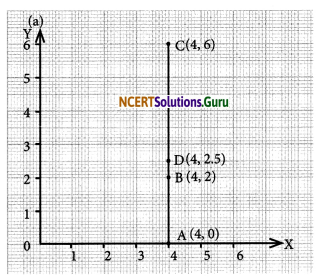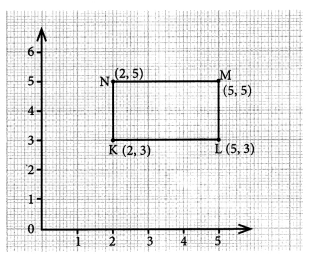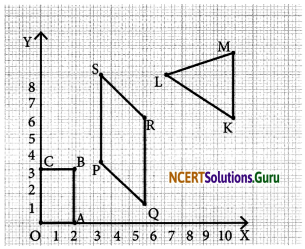# NCERT Solutions for Class 8 Maths Chapter 15 Introduction to Graphs Ex 15.2

These NCERT Solutions for Class 8 Maths Chapter 15 Introduction to Graphs Ex 15.2 Questions and Answers are prepared by our highly skilled subject experts.

## NCERT Solutions for Class 8 Maths Chapter 15 Introduction to Graphs Exercise 15.2

Question 1.
Plot the following points on a graph sheet. Verify if they lie on a line
(a) A (4,0), B (4, 2), C(4, 6), D(4, 2.5)
(b) P(1, 1),Q(2,2),R(3,3),S(4,4)
(c) K(2, 3) L(5, 3), M(5, 5), N(2, 5)
In each case we draw the x-axis and the y-axis and plot the given points.
(a) On plotting the points A (4,0) B (4,2) C(4, 6) and D(4, 2.5) and then on joining them we find that they lie on the same line.(b) On plotting the points P(l, 1), Q(2, 2), R(3, 3) and S(4, 4) and then on joining them we find that they all lie on the same line.(c) Plotting the points K(2, 3), L(5, 3), M(5, 5) and N(2, 5) and on joining them we find that all of them do not lie on the same line.Question 2.
Draw the line passing through (2,3) and (3,2). Find the coordinates of the points at which this line meets the x-axis and y-axis.
On plotting the points A (2, 3) and B(3, 2), we draw line that joins them. On extending, the line meets the x-axis at C(5,0) and the y-axis at D(0, 5).Question 3.
Write the coordinates of the vertices of each of these adjoining figures.(i) The coordinates of‘O’ are (0,0), A are (2,0), B are (2, 3) and C are (0, 3)
(ii) The coordinate of P are (4, 3), Q are (6, 1), R are (6, 5), S are (4, 7)
(iii) The coordinate of K are (10, 5), L are (7, 7) and M are (10, 8)

Question 4.
State whether True or False. Correct if they are false.
(i) A point whose x-coordinate is zero and y-coordinate is non-zero will lie on the y-axis.
(ii) A point whose y-coordinate is zero and x-coordinate is 5 will lie on y-axis.
(iii) The coordinates of the origin are (0,0).